# Banach limit

Banach limits originated in [a1], Chapt. II, Sect. 3. Denoting the positive integers by, the set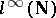is the real vector space of all bounded sequences of real numbers. For any element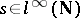, one defines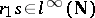by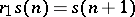for all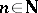. S. Banach showed that there exists an element in the dual, called, such that

1)for all;

2)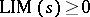for all non-negative sequences;

3)for all;

4)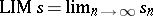for all convergent sequences. Banach proved the existence of this generalized limit by using the Hahn–Banach theorem. Today (1996), Banach limits are studied via the notion of amenability.

For a semi-groupone defines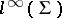to be the real vector space of all real bounded functions on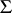. For an element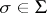one denotes the left (respectively, right) shift by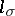(respectively,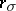). Thus,for all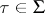and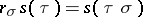for all. An elementis called a left- (respectively right-) invariant mean if

1);

2)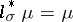(respectively,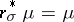), where, e.g.,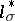is the adjoint of.itself is called left (respectively, right) amenable if there exists a left- (respectively, right-) invariant mean in. The existence of Banach limits above is a special case of an invariant mean, whereequals the semi-group of natural numbers. Banach also proved that the real numbers are amenable (left and right). M.M. Day has proved that every Abelian semi-group is left and right amenable. On the other hand,, the free group on two generators, is not amenable.

Another approach to amenability is the measure-theoretic point of view. In fact, the prehistory of amenability starts with the following question by H. Lebesgue in the classic "Leçons sur L'Intégration et la Recherche des Fonctions Primitives" ([a5], pp. 114–115): Can countable additivity of the Lebesgue measure be replaced by finite additivity? Banach answered the question in the negative, constructing a finitely additive measure on all subsets of the real numbers, invariant under translation, again using the Hahn–Banach theorem. More generally, if a groupis acting on a set, a finitely additive probability measure on the collection of all subsets of, invariant under, is sometimes also called an invariant mean. If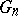is the isometry group of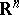, one can ask for a finitely additive measure invariant under. Such a measure does exist for, but not for. Forthis leads to so-called paradoxical decompositions or the Banach–Tarski paradox (see Tarski problem; for a survey, see [a8]). For all, the groupcontains the non-amenable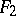as a subgroup. It has been proved that the Banach–Tarski paradox is effectively (i.e., in ZF set theory) implied by the Hahn–Banach theorem (see [a7]).

For a survey of results of the role of amenability, see [a6] and for a survey of the Hahn–Banach theorem, see [a2]. For the early history of Banach limits and invariant means, including many important results, see [a3] and [a4].

How to Cite This Entry:
Banach limit. Encyclopedia of Mathematics. URL: http://encyclopediaofmath.org/index.php?title=Banach_limit&oldid=39373
This article was adapted from an original article by G. Buskes (originator), which appeared in Encyclopedia of Mathematics - ISBN 1402006098. See original article